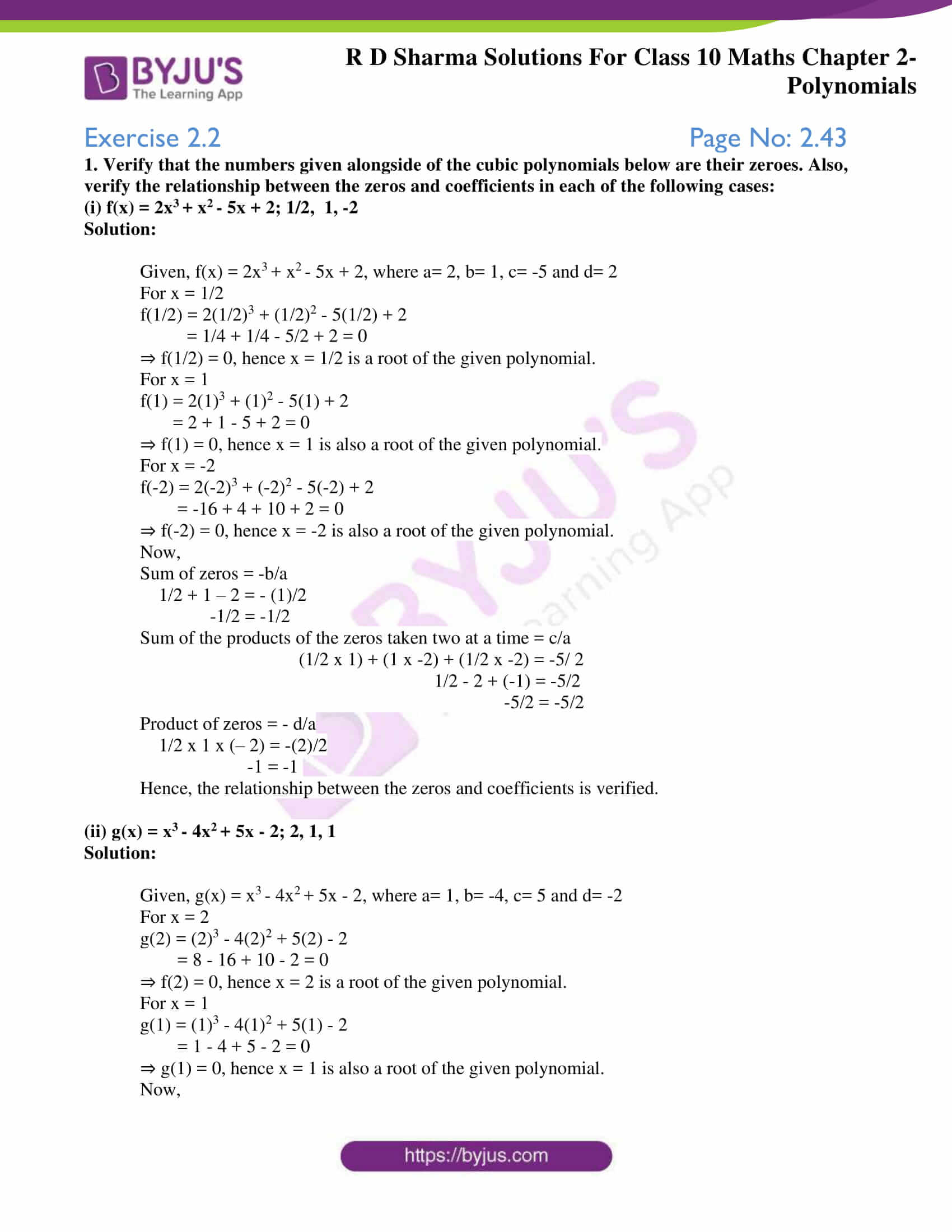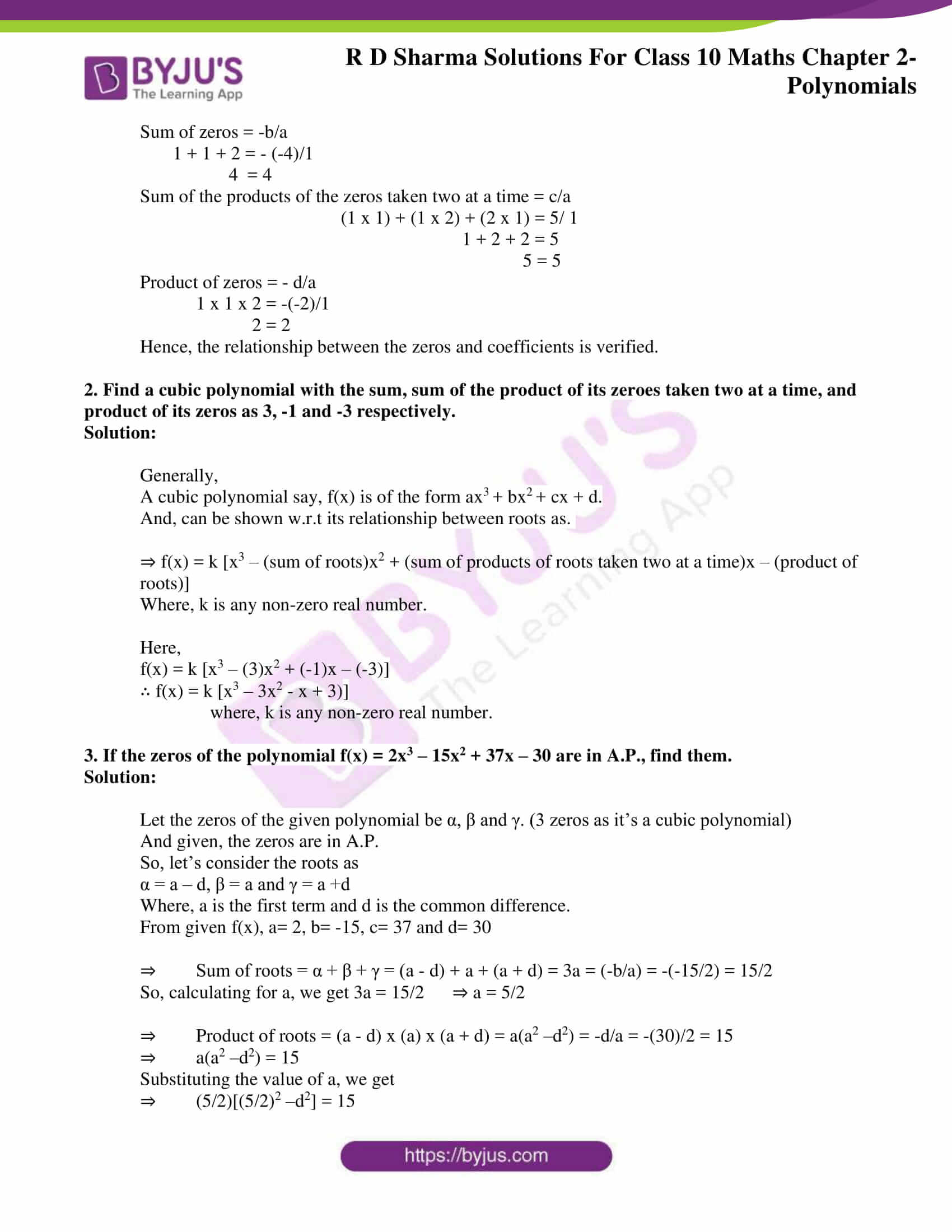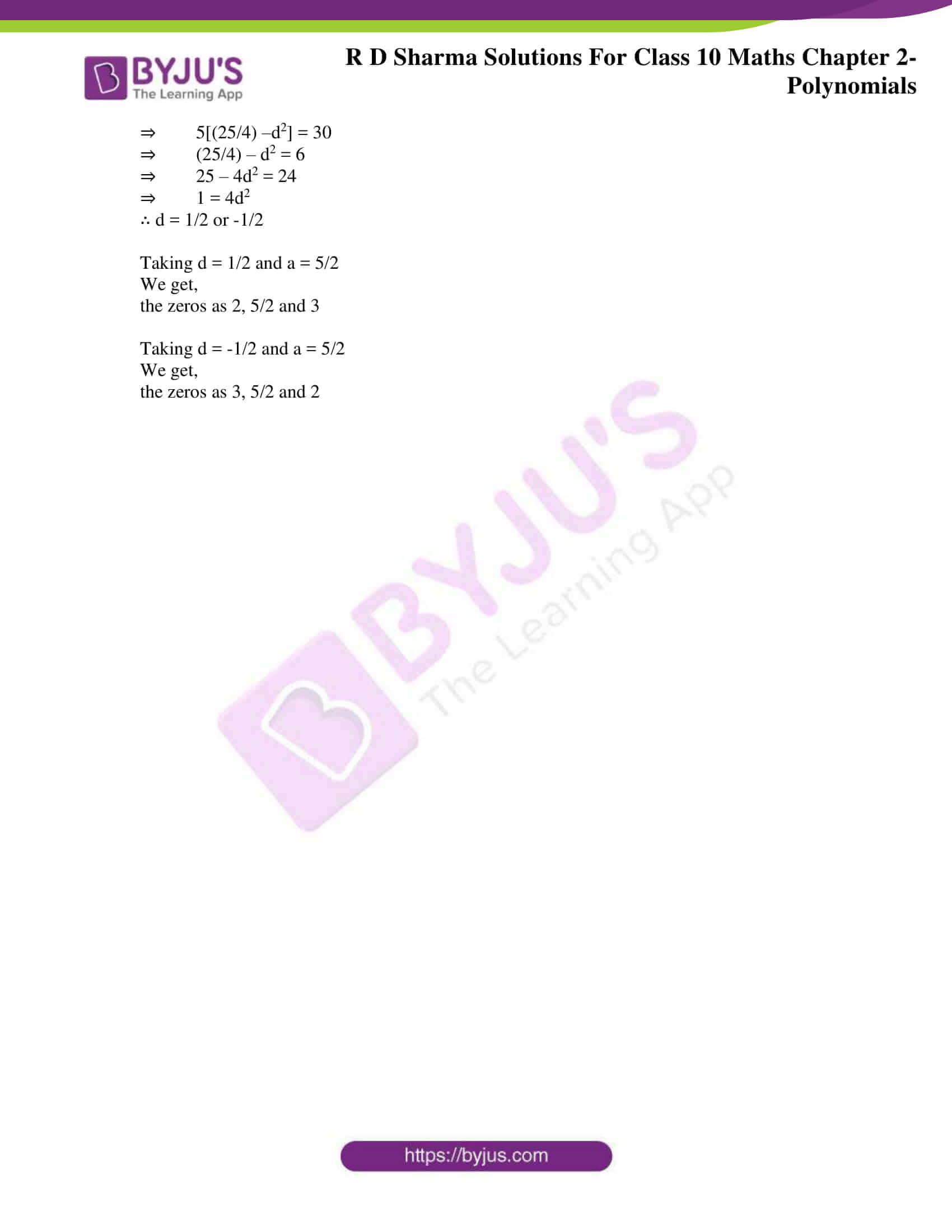This exercise majorly focuses on verifying the zeros and the relationship between the zeros and coefficients of a cubic polynomial. The RD Sharma Solutions Class 10 prepared by experts at BYJU’S can help students understand these concepts clearly following the latest CBSE patterns. As a result, the students can achieve higher marks in their examinations. Students can download RD Sharma Solutions for Class 10 Maths Chapter 2 Polynomials Exercise 2.2 PDF provided below for a detailed explanation of problems in this exercise.

## RD Sharma Solutions for Class 10 Maths Chapter 2 Polynomials Exercise 2.2 Download PDF### Access RD Sharma Solutions for Class 10 Maths Chapter 2 Polynomials Exercise 2.2

1. Verify that the numbers given alongside of the cubic polynomials below are their zeroes. Also, verify the relationship between the zeros and coefficients in each of the following cases:

(i) f(x) = 2x3 + x2 – 5x + 2; 1/2, 1, -2

Solution:

Given, f(x) = 2x3 + x2 – 5x + 2, where a= 2, b= 1, c= -5 and d= 2

For x = 1/2

f(1/2) = 2(1/2)3 + (1/2)2 – 5(1/2) + 2

= 1/4 + 1/4 – 5/2 + 2 = 0

⇒ f(1/2) = 0, hence x = 1/2 is a root of the given polynomial.

For x = 1

f(1) = 2(1)3 + (1)2 – 5(1) + 2

= 2 + 1 – 5 + 2 = 0

⇒ f(1) = 0, hence x = 1 is also a root of the given polynomial.

For x = -2

f(-2) = 2(-2)3 + (-2)2 – 5(-2) + 2

= -16 + 4 + 10 + 2 = 0

⇒ f(-2) = 0, hence x = -2 is also a root of the given polynomial.

Now,

Sum of zeros = -b/a

1/2 + 1 – 2 = – (1)/2

-1/2 = -1/2

Sum of the products of the zeros taken two at a time = c/a

(1/2 x 1) + (1 x -2) + (1/2 x -2) = -5/ 2

1/2 – 2 + (-1) = -5/2

-5/2 = -5/2

Product of zeros = – d/a

1/2 x 1 x (– 2) = -(2)/2

-1 = -1

Hence, the relationship between the zeros and coefficients is verified.

(ii) g(x) = x3 – 4x2 + 5x – 2; 2, 1, 1

Solution:

Given, g(x) = x3 – 4x2 + 5x – 2, where a= 1, b= -4, c= 5 and d= -2

For x = 2

g(2) = (2)3 – 4(2)2 + 5(2) – 2

= 8 – 16 + 10 – 2 = 0

⇒ f(2) = 0, hence x = 2 is a root of the given polynomial.

For x = 1

g(1) = (1)3 – 4(1)2 + 5(1) – 2

= 1 – 4 + 5 – 2 = 0

⇒ g(1) = 0, hence x = 1 is also a root of the given polynomial.

Now,

Sum of zeros = -b/a

1 + 1 + 2 = – (-4)/1

4 = 4

Sum of the products of the zeros taken two at a time = c/a

(1 x 1) + (1 x 2) + (2 x 1) = 5/ 1

1 + 2 + 2 = 5

5 = 5

Product of zeros = – d/a

1 x 1 x 2 = -(-2)/1

2 = 2

Hence, the relationship between the zeros and coefficients is verified.

2. Find a cubic polynomial with the sum, sum of the product of its zeroes taken two at a time, and product of its zeros as 3, -1 and -3 respectively.

Solution:

Generally,

A cubic polynomial say, f(x) is of the form ax3 + bx2 + cx + d.

And, can be shown w.r.t its relationship between roots as.

⇒ f(x) = k [x3 – (sum of roots)x2 + (sum of products of roots taken two at a time)x – (product of roots)]

Where, k is any non-zero real number.

Here,

f(x) = k [x3 – (3)x2 + (-1)x – (-3)]

∴ f(x) = k [x3 – 3x2 – x + 3)]

where, k is any non-zero real number.

3. If the zeros of the polynomial f(x) = 2x3 – 15x2 + 37x – 30 are in A.P., find them.

Solution:

Let the zeros of the given polynomial be α, β and γ. (3 zeros as it’s a cubic polynomial)

And given, the zeros are in A.P.

So, let’s consider the roots as

α = a – d, β = a and γ = a +d

Where, a is the first term and d is the common difference.

From given f(x), a= 2, b= -15, c= 37 and d= 30

⇒ Sum of roots = α + β + γ = (a – d) + a + (a + d) = 3a = (-b/a) = -(-15/2) = 15/2

So, calculating for a, we get 3a = 15/2 ⇒ a = 5/2

⇒ Product of roots = (a – d) x (a) x (a + d) = a(a2 –d2) = -d/a = -(30)/2 = 15

⇒ a(a2 –d2) = 15

Substituting the value of a, we get

⇒ (5/2)[(5/2)2 –d2] = 15

⇒ 5[(25/4) –d2] = 30

⇒ (25/4) – d2 = 6

⇒ 25 – 4d2 = 24

⇒ 1 = 4d2

∴ d = 1/2 or -1/2

Taking d = 1/2 and a = 5/2

We get,

the zeros as 2, 5/2 and 3

Taking d = -1/2 and a = 5/2

We get,

the zeros as 3, 5/2 and 2

1. Good